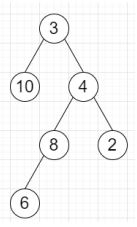# Program to find an ancestor which is common of two elements in a binary tree in Python

Suppose we have a binary tree, and we also have two numbers a and b, we have to find the value of the lowest node that has a and b as descendants. We have to keep in mind that a node can be a descendant of itself.

So, if the input is likea = 6, b = 2, then the output will be 4

To solve this, we will follow these steps −

• Define a method solve() this will take root and a, b

• if root is null, then

• return -1

• if value of root is either a or b, then

• return value of root

• left := solve(left of root, a, b)

• right := solve(right of root, a, b)

• if left or right is not -1, then

• return value of root

• return left if left is not same as -1 otherwise right

• from the main method call solve(root)

Let us see the following implementation to get better understanding −

## Example

Live Demo

class TreeNode:
def __init__(self, val, left=None, right=None):
self.val = val
self.left = left
self.right = right

class Solution:
def solve(self, root, a, b):
if not root:
return -1
if root.val in (a, b):
return root.val
left = self.solve(root.left, a, b)
right = self.solve(root.right, a, b)
if -1 not in (left, right):
return root.val
return left if left != -1 else right
ob = Solution()
root = TreeNode(3)
root.left = TreeNode(10)
root.right = TreeNode(4)
root.right.left = TreeNode(8)
root.right.right = TreeNode(2)
root.right.left.left = TreeNode(6)
print(ob.solve(root, 6, 2))

## Input

root = TreeNode(3)
root.left = TreeNode(10)
root.right = TreeNode(4)
root.right.left = TreeNode(8)
root.right.right = TreeNode(2)
root.right.left.left = TreeNode(6)
6, 2

## Output

4

Updated on: 12-Oct-2020

135 Views